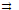Hybridization

Atomic orbitals can be combined, in a process called hybridization, to describe the bonding in polyatomic molecules.

Descriptions of the bonding in CH4 can be used to illustrate the valence bond procedure. We must arrive at four bonds projecting from the carbon atom in procedure. We must arrive at four bonds projecting from the carbon atom in tetrahedral directions.

Lithus Pauling pointed out that the 2s and 3p orbitals of the carbon atom could be used to form new orbitals better suited to the description of the bonds. This procedure of combining orbitals to form new ones is called hybridization, and the new sets are called hybrid orbitals. The most suitable set can be found, according to Pauling, by forming wave functions which project out farthest from the central atom. When the four orbitals that they are concentrated along tetrahedral directions. Thus the sp3 hybrid orbitals are tetrahedrally oriented and are suitable for describing the bonding in CH4.

Other combinations of s, p and d orbitals can be constructed to provide orbitals suitable for molecules of other shapes, hybrid orbitals that project in linear, trigonal, tetrahedral and octahedral directions are produced by the combinations. The trigonal and linear hybrids, which leave one p and two p orbitals of the atom unchanged, are the basis for descriptions of double and triple bonds. The p orbitals form bonds and supplement the σ bonds, to notice that σ and bonds are similar to those constructed for homonuclear diatomic molecules.

Hybrid orbitals from symmetry: the hybrid orbitals constructed by Pauling led to the geometry, or symmetry, of the molecule for which they were constructed. If the geometry of the molecule is taken as known, the approximate hybrid orbitals can be deduced from symmetry consideration alone. Consider the four tetrahedrally arranged carbon atom bond orbitals needed in this approach to describe the bonding in methane. For these orbitals the characters for the various symmetry operations of the Td group can be seen by calculating the number of unchanged bond orbitals, or bond lines, for each operation. We obtain:

 Td E 8C3 3C2 6σd 6S4 σorb 4 1 0 2 0

Thus we need atomic orbitals that transform as A1 and T2 to provide the basis for the tetrahedrally directed hybrid orbitals. The totally symmetric s atomic orbital transforms according to A1. In a similar way, the hybrid combinations of table can be deduced from the symmetry of the bonding situation for which they are to be used.

Some Hybridization used in describing σ bonding:

 Number of orbitals Shape Atomic-Orbital Combinations Example 2 Linear sp CH≡CH 3 Trigonal sp2 CH2CH2, BF3 4 Tetrahedral sp3 or sd3 CH4, MnO4- Square planner dsp2 PtCl24-, Ni(CN)24- 5 Trigonal bipyramid dsp3 PCl5, Fe (CO)5 6 Octahedron d2sp3 PtF6, CoF26-

#### Related Questions in Chemistry

• ##### Q :Calculating density of water using

What is the percent error in calculating the density of water using the ideal gas law for the following conditions:  a. 110 oC, 1 bar   b. 210 oC 10 bar  c. 374 o

• ##### Q :Ionic radius of chloride ion The edge

The edge length of the unit cell of Nacl crystal lattice is 552 pm. If ionic radius of sodium ion is 95. What is the ionic radius of chloride ion:(a) 190 pm  (b) 368 pm  (c) 181 pm  (d) 276 pm     <

• ##### Q :Problem on molality Select the right

Select the right answer of the question. Calculate the molality of 1 litre solution of 93% H2SO4 (weight/volume). The density of the solution is 1.84 g /ml : (a) 10.43 (b) 20.36 (c) 12.05 (d) 14.05

• ##### Q :Lab question Explain how dissolving the

Explain how dissolving the Group IV carbonate precipitate with 6M CH3COOH, followed by the addition of extra acetic acid, establishes a buffer with a pH of approximately 5.

• ##### Q :Q what is the basicity of primary

what is the basicity of primary secondary and tertiary amines in chlorobenzene

• ##### Q :Define Bond Energies - Bond Charges

Energy changes in some chemical reactions can be used to deduce the energies of chemical bonds. Our understanding of the molecular basis of thermodynamic properties is extended when we ask why the enthalpy change for a reaction is what it is. We deduce,

• ##### Q :Problem based on normality Choose the

Choose the right answer from following. NaClO solution reacts with H2SO3 as,. NaClO + H2SO3→NaCl+ H2SO4. A solution of NaClO utilized in the above reaction contained 15g of NaClO per litre. The

• ##### Q :Value of molar solution Select the

Select the right answer of the question. Molar solution contains: (a)1000g of solute (b)1000g of solvent (c)1 litre of solvent (d)1 litre of solution

• ##### Q :Molarity of pure water Choose the right

Choose the right answer from following. The molarity of pure water is: (a) 55.6 (b) 5.56 (c)100 (d)18

• ##### Q :Mass percent Help me to go through this

Help me to go through this problem. 10 grams of a solute is dissolved in 90 grams of a solvent. Its mass percent in solution is : (a) 0.01 (b) 11.1 (c)10 (d) 9